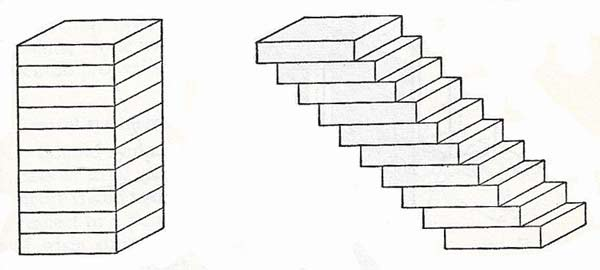A# Cavalieri's principleCavalieri's principle, named after the Italian mathematician Bonaventuri Cavalieri (1598–1647), states that if two solid bodies are of equal height and have bases of equal area, and if all plane sections parallel to the bases and at the same distances from the corresponding bases are equal in area, then the two solids are equal in volume. Cavalieri's theorem cannot be proved by the methods of elementary mathematics, but the figures shown here make the proposition plausible